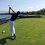# Expected sight distance on earth

Consider the earth as the perfect spheroid

$\frac{x^2}{400^2}+\frac{y^2}{400^2}+\frac{z^2}{100^2}=1$

and assume it's surface to be completely land. A person whose eyes are 2 meters above the ground is randomly placed on the earth facing a random direction. What is the expected sight distance of this person?

Sight distance is defined as follows.

A ray is drawn from the person's eyes in the direction he/she is facing such that it is tangent to the spheroid at point B. The linear distance from the person's eyes to point B is the sight distance.

I just thought of this problem today and I have no clue how difficult it is. Approximations are welcomed (for example, calculating the expected distance from the person's feet to point B.Note by Trevor Arashiro
4 years, 5 months ago

This discussion board is a place to discuss our Daily Challenges and the math and science related to those challenges. Explanations are more than just a solution — they should explain the steps and thinking strategies that you used to obtain the solution. Comments should further the discussion of math and science.

When posting on Brilliant:

• Use the emojis to react to an explanation, whether you're congratulating a job well done , or just really confused .
• Ask specific questions about the challenge or the steps in somebody's explanation. Well-posed questions can add a lot to the discussion, but posting "I don't understand!" doesn't help anyone.
• Try to contribute something new to the discussion, whether it is an extension, generalization or other idea related to the challenge.

MarkdownAppears as
*italics* or _italics_ italics
**bold** or __bold__ bold
- bulleted- list
• bulleted
• list
1. numbered2. list
1. numbered
2. list
Note: you must add a full line of space before and after lists for them to show up correctly
paragraph 1paragraph 2

paragraph 1

paragraph 2

[example link](https://brilliant.org)example link
> This is a quote
This is a quote
    # I indented these lines
# 4 spaces, and now they show
# up as a code block.

print "hello world"
# I indented these lines
# 4 spaces, and now they show
# up as a code block.

print "hello world"
MathAppears as
Remember to wrap math in $$ ... $$ or $ ... $ to ensure proper formatting.
2 \times 3 $2 \times 3$
2^{34} $2^{34}$
a_{i-1} $a_{i-1}$
\frac{2}{3} $\frac{2}{3}$
\sqrt{2} $\sqrt{2}$
\sum_{i=1}^3 $\sum_{i=1}^3$
\sin \theta $\sin \theta$
\boxed{123} $\boxed{123}$

Sort by:

How about we try a Monte-Carlo Simulation?

- 4 years, 4 months ago

Well, isn't it similar to the method by which we determine the height of any transmission tower (to be used for communication purposes)? There, we draw a tangent to the surface of the earth and then calculate the height of the tower using basic trigonometry and approximations. Here, we have to go in reverse order. Correct me if I'm wrong.

- 4 years, 2 months ago

Yes, we only have to draw tangents, and that's easy if you assume that the earth is a sphere because all tangents will be of the same length. However, on a spheroid, it's much more difficult.

- 4 years, 1 month ago

It might be good to build this one up one piece at a time. For example, we could start with the following problem:

Given a certain spheroid and a particular point in space, what is the shortest / longest tangent line segment from the point to the spheroid?

I think that would be a fun one to post as a problem.

- 4 years ago

Yes, this sounds promising.

How do we solve the first problem?

Yep, got it. Thanks for pointing out.

- 4 years ago